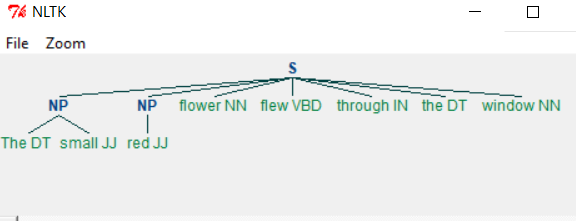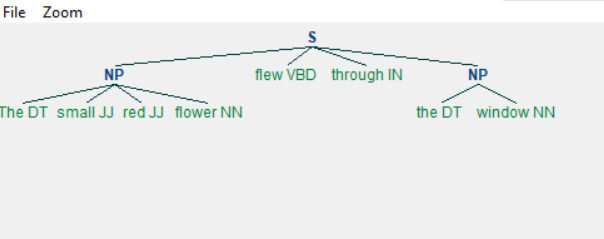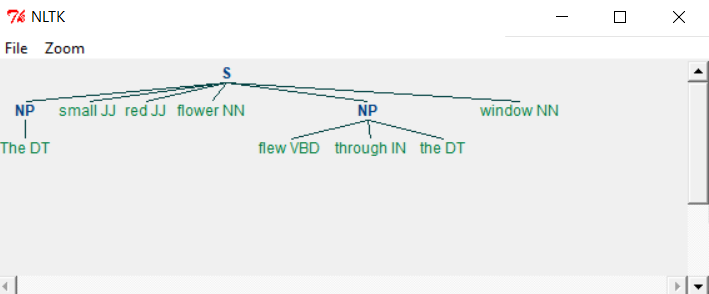# Python – Chunks and Chinks

Chunking is the process of grouping similar words together based on the nature of the word. In the below example we define a grammar by which the chunk must be generated. The grammar suggests the sequence of the phrases like nouns and adjectives etc. which will be followed when creating the chunks. The pictorial output of chunks is shown below.

```import nltk

sentence = [("The", "DT"), ("small", "JJ"), ("red", "JJ"),("flower", "NN"),
("flew", "VBD"), ("through", "IN"),  ("the", "DT"), ("window", "NN")]
grammar = "NP: {?*}"
cp = nltk.RegexpParser(grammar)
result = cp.parse(sentence)
print(result)
result.draw()
```

When we run the above program we get the following output −Changing the grammar, we get a different output as shown below.

```import nltk

sentence = [("The", "DT"), ("small", "JJ"), ("red", "JJ"),("flower", "NN"),
("flew", "VBD"), ("through", "IN"),  ("the", "DT"), ("window", "NN")]

grammar = "NP: {?*}"

chunkprofile = nltk.RegexpParser(grammar)
result = chunkprofile.parse(sentence)
print(result)
result.draw()
```

When we run the above program we get the following output −## Chinking

Chinking is the process of removing a sequence of tokens from a chunk. If the sequence of tokens appears in the middle of the chunk, these tokens are removed, leaving two chunks where they were already present.

```import nltk

sentence = [("The", "DT"), ("small", "JJ"), ("red", "JJ"),("flower", "NN"), ("flew", "VBD"), ("through", "IN"),  ("the", "DT"), ("window", "NN")]

grammar = r"""
NP:
{<.*>+}         # Chunk everything
}+{      # Chink sequences of JJ and NN
"""
chunkprofile = nltk.RegexpParser(grammar)
result = chunkprofile.parse(sentence)
print(result)
result.draw()```

When we run the above program, we get the following output −As you can see the parts meeting the criteria in grammar are left out from the Noun phrases as separate chunks. This process of extracting text not in the required chunk is called chinking.